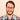# Comparing two images using NumPy and pillow

Lately, I've been contributing to Pyscript in my free time. Most of my contributions have been tackling the epic to improve test coverage. I also find it helpful to start looking at or creating tests when getting started on a new project.

Pyscript has a lot of examples in the repository, and the tests use these for integration tests. One of the examples uses NumPy and Matplotlib to generate a graph (see the example). Initially, I had no idea how to test this since the page renders the graph in an `img` tag.

Luckily, Madhur Tandon pointed me in the right direction. The suggestion:

One approach could be to compare the underlying numpy data for the image rendered through the canvas along with a reference image uploaded to the repository.

Github Review

In this article, I will describe how I wrote the test to confirm that the two images are the same by using NumPy. Although, all credits go to Madhur and the code you can find in the matplotlib_pyodide package.

## The Requirements

Here's all we need to test that the two images are the same:

We will use Pillow to create the image from bytes and then NumPy to confirm that both images are identical. This

We need an image to use as a reference because the matplotlib example generates the graph each time the example is run. We want to ensure that if breaking changes are introduced that cause the image to be different, we will know immediately by the test failure.

## The Test

The graph produced by Matplotlib is being added to the page by passing its base64 encoded string as the source. Since we have the base64 encoded string in the source of the image, we can get the image and then read its `src` attribute.

Pyscript uses Playwright for the integration tests, so we can use playwright to fetch the image source. Also, this page contains a single image, so we don't need to worry about being specific about which to grab.

`python`1# First get image from the page and then get the src details2img_src = self.page.wait_for_selector("img").get_attribute("src")3# Replace anything that is not the base64 string4img_src = img_src.replace("data:image/png;charset=utf-8;base64,", "")5# Finally, decode the base64 string to get the image bytes6img_bytes = base64.b64decode(img_src)``

We need to recreate the image from its bytes and generate a NumPy array from it.

`python`1import io2import numpy as np3from PIL import Image4# Recreate image using pillow5img = Image.open(io.BytesIO(img_bytes))6# Generate the numpy array7img_data = np.asarray(img)``

### Create the NumPy array from the reference image

Now we need to do the same for our reference image. Since we have the image stored, we can open it with Pillow and generate the NumPy array. If you are unfamiliar with Pillow, this library allows you to open images directly, so you don't need to open them as bytes first.

`python`1import os2dir = os.path.dirname(__file__)3with Image.open(os.path.join(dir, "test_assets", "tripcolor.png")) as image:4  ref_data = np.asarray(image)``

## Comparing the two images

We now have the representation of the two images as a Numpy array. We can compare both images by subtracting both arrays and get the mean. If both images are the same, then the result of the subtraction will be an array filled with zeros, and the mean returned should be 0.0

`python`1deviation = np.mean(np.abs(img_data - ref_data))2# Confirm that both are the same image - should return 0.03assert deviation == 0.0``

That's all there is to it. Again let me reiterate that this code came from the pyodide matplotlib package.

## The whole code

Here's the whole code in case you need it - note that it contains some testing machinery that Pyscript uses.

```python```1import io2import numpy as np3from PIL import Image4
5
6def test_matplotlib(self):7    self.goto("examples/matplotlib.html")8    self.wait_for_pyscript()9    assert self.page.title() == "Matplotlib"10    wait_for_render(self.page, "*", "<img src=['\"]data:image")11    # The image is being rended using base64, lets fetch its source12    # and replace everything but the actual base64 string.\13    img_src = self.page.wait_for_selector("img").get_attribute("src").replace("data:image/png;charset=utf-8;base64,", "")14    # Finally, let's  get the np array from the previous data15    img_data = np.asarray(Image.open(io.BytesIO(base64.b64decode(img_src))))16    with Image.open(17        os.path.join(os.path.dirname(__file__), "test_assets", "tripcolor.png"),18    ) as image:19        ref_data = np.asarray(image)20    # Now that we have both images data as a numpy array21    # let's confirm that they are the same22    deviation = np.mean(np.abs(img_data - ref_data))23    assert deviation == 0.0``````

References:

0 Like 0 Comment

### You might also like these